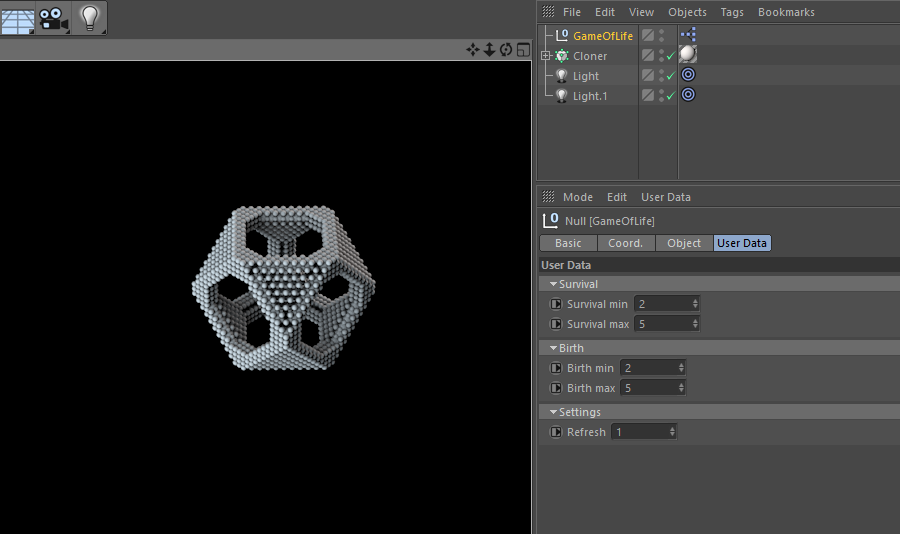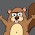## 01 January 2018

### [C4D Script] Game of life

Another programming classic : Game Of Life (Cellular Automata family).

Game of life is not a game and has nothing to do with life. but where it gets interesting, is in the fact that you can build complexe patterns whith different behaviours only with very simple rules.

The original Game of life rules (for a 2D space) are:

• Each cell with one or no neighbors dies, as if by solitude.
• Each cell with more than three neighbors dies, as if by overpopulation.
• Each cell with two or three neighbors survives.
• Any dead cell with exactly three live neighbours becomes a live cell, as if by reproduction.

In this script (Xpresso python node) I tried to create 3D version of Game of life with the possibility to have dynamic rules. You can start with some rules and change them right in the middle of your simulation.

It's a is time based animation. Every frame, new calculations are done and there is no bounderies for your final shape. as far as your rules allows expansion of your pattern and your PC can handle it :)

To create your own simulation, you have to :

1. Define a set of starting points (see fill_grid method in source code)
2. Set up your simulation rules
3. Press play button to start simulation### Source code

``````
import c4d
from c4d import documents, Vector
from collections import defaultdict

class MultidimensionalDictionary:

def __init__(self):
self.cells = defaultdict(lambda: defaultdict(dict))

def get_value(self, i, j, k):
if self.cells.has_key(i) and self.cells[i].has_key(j) and self.cells[i][j].has_key(k):
return self.cells[i][j][k]
return None

def set_value(self, i, j, k, value):
self.cells[i][j][k] = value
return self

def for_each(self, fn):
for ikey, ivalue in self.cells.items():
for jkey, jvalue in ivalue.items():
for kkey, kvalue in jvalue.items():
fn(kvalue, ikey, jkey, kkey)

class Helper:

@staticmethod
def create_dict():
return defaultdict(lambda: defaultdict(dict))

@staticmethod
def for_each(array, fn):
for value in array:
fn(value)

@staticmethod
def loop_around(i, j, k, fn):
for a in xrange(i - 1, i + 2):
for b in xrange(j - 1, j + 2):
for c in xrange(k - 1, k + 2):
if (a, b, c) != (i, j, k):
fn(a, b, c)

@staticmethod
def fill_grid(generation):
"""
"""
init_points = [(-1, 0, 0), (1, 0, 0), (0, 0, 1), (0, 0, -1), (0, 1, 0), (0, -1, 0)]

for p in init_points:
generation.grid.set_value(p, p, p, Cell())

class Cell:

def __init__(self, age=1):
self.age = age

class Generation:

def __init__(self):
self.grid = MultidimensionalDictionary()

def get_cells_count_around(self, i, j, k):
counter = 0
for a in xrange(i - 1, i + 2):
for b in xrange(j - 1, j + 2):
for c in xrange(k - 1, k + 2):
if self.grid.get_value(a, b, c):
counter += 1

return counter - 1 if self.grid.get_value(i, j, k) else counter

def get_next_generation(self):
""" Get next cells generation """

new_generation = Generation()

def birth(i, j, k):
""" Checks if a new cell can be born """
current_cell = self.grid.get_value(i, j, k)
if current_cell is None:
count = self.get_cells_count_around(i, j, k)
if birth_min <= count <= birth_max:
new_generation.grid.set_value(i, j, k, Cell())

def update_cell(selected_cell, i, j, k):
""" Checks if the current cell can survive """
count = self.get_cells_count_around(i, j, k)
if survival_min <= count <= survival_max:
selected_cell.age += 1
new_generation.grid.set_value(i, j, k, selected_cell)
Helper.loop_around(i, j, k, birth)

self.grid.for_each(update_cell)
return new_generation

class C4dRender:

SCALE_COEF = 1
OLDEST_CELL_AGE = 0

def __init__(self):
self.generation = Generation()
self.doc = documents.GetActiveDocument()
self.tp = self.doc.GetParticleSystem()
self.root_group = self.tp.GetRootGroup()

Helper.fill_grid(self.generation)

def update(self):
self.tp.FreeAllParticles()
self.generation.grid.for_each(self.render)
self.generation = self.generation.get_next_generation()

def render(self, cell, i, j, k):
C4dRender.OLDEST_CELL_AGE = cell.age if cell.age > C4dRender.OLDEST_CELL_AGE else C4dRender.OLDEST_CELL_AGE
# drawing particle
p = self.tp.AllocParticle()
self.tp.SetGroup(p, self.root_group)
self.tp.SetPosition(p, Vector(i, j, k) * C4dRender.SCALE_COEF)
self.tp.SetSize(p, cell.age * 0.5)
self.tp.SetColor(p, Vector(c4d.utils.RangeMap(cell.age, 0, C4dRender.OLDEST_CELL_AGE, 0, 1, True), 0.2, 0.4))

instance = C4dRender()

def main():

if frame % refresh == 0:
instance.update()
``````

1.How to add starting points ????

1.Hi! You have to edit the GameOFLife script like this :
- Go to the fill_grid method
- Edit the init points list by setting your custom points. each item/tuple of this list represents a point position with its three components (x,y, z)

Now you can run your animation and ... voila :)

2.Thank you. I was trying it in R14, did not work there.

1.Hi,
This is due to the use of an unimplemented function in the API of Cinema4D R14, which is: setColor().
You can fix it easily by adding a hash symbol # at the beginging of the line 129, or even deleting it.

Here is the concerned line (129):
self.tp.SetColor(p, Vector(c4d.utils.RangeMap(cell.age, 0, C4dRender.OLDEST_CELL_AGE, 0, 1, True), 0.2, 0.4))

3.WOW.. that worked like a charm, thank you very much...Very well made. my hat's off to you

1.you're welcome :)